Application Note

# Better metrics for comparing instruments and assays

### Introduction

When comparing instruments, one must choose a metric by which to measure their performance. Typically, one is interested in how “sensitive” an instrument is, but sensitivity can be measured in many different ways. Metrics used to measure sensitivity include signal-to-background ratio (S/B), signal-to-noise ratio (S/N), lower limit of detection (LLD) and Z’-factor1.

Fundamentally, one is interested in whether or not an instrument can detect a signal for a given assay; that is, whether a signal is detectable above the background of the instrument. Being able to detect a signal above background depends not just on the mean signal and background levels, but also on other factors such as the variation in the signal and the background.

To understand this better, consider the analogy of looking for a 1.9 m tall friend in a crowd:

• If everyone else in the crowd is 1.6 m tall, then the mean background is uniform and lower than the signal (the friend), so the friend is clearly visible.
• If everyone else in the crowd is 1.9 m tall, then the signal is the same as the mean background. Although the background noise is low, the mean background is high so the friend is not visible.
• Finally, if the people in the crowd range in height equally distributed from 1.5 to 2 m tall, the mean background is 1.75 m. The mean background is lower than the signal, but the variation in height (i.e. the noise) will make it difficult to see the friend.

Thus, the signal, the mean background, and the background variation must all be considered when determining detection limits.

If we generalize the analogy to take into account a group (population) of friends, then the variation in the signal also becomes important (it is easier to find a number of friends when they are all the same height, or if they vary in height, when they are all taller than the crowd).

Thus we have identified the important parameters when assessing metrics for comparing instruments. The ideal metric will consider the following:

• Mean signal
• Signal variation
• Mean background
• Background variation

### Signal-to-Background Ratio

Signal-to-background ratio is the ratio of the mean signal level to the mean background level: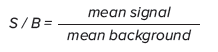As discussed above, the signal-to-background ratio is inadequate as a measure of sensitivity because it does not include any information about signal variation or background variation2 (see also Figure 1 and Figure 2 below). Signal-to-background does not provide a means of distinguishing between two instruments, one having high background variability and the other having low background variability, even though their mean background is the same.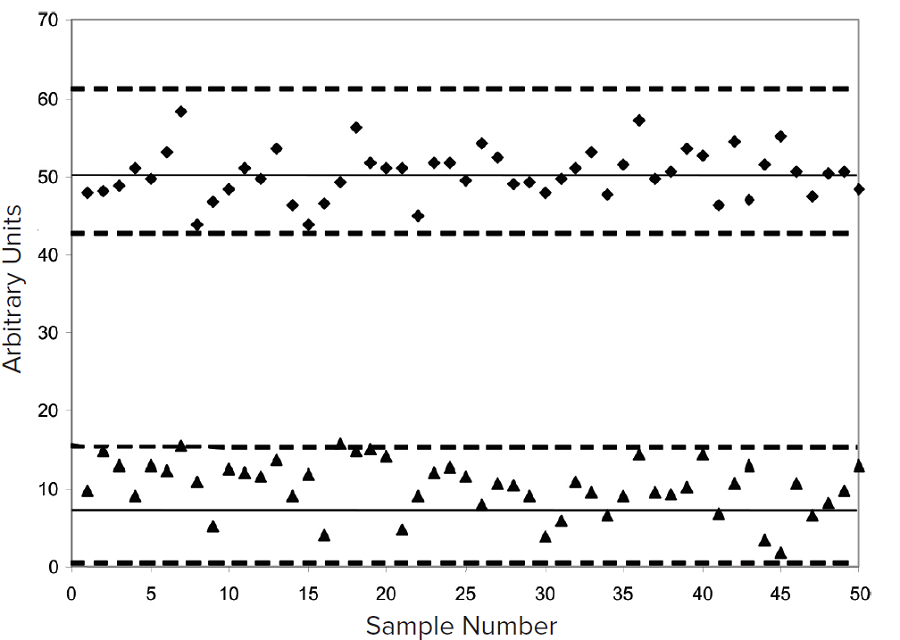Figure 1. Signal and background, higher background variation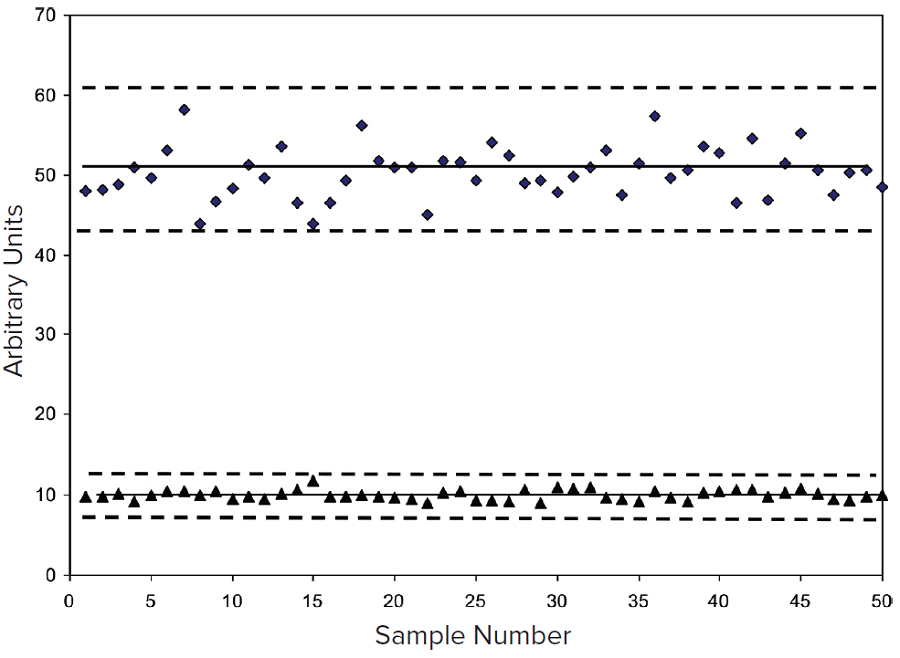Figure 2. Signal and background, lower background variation

### Signal-to-Noise Ratio

Signal-to-noise ratio is a better metric than signal-to-background because it does take into account variation in the background: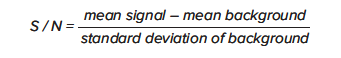Signal-to-noise ratio is an important and widely used metric for determining the confidence with which one can quantify a signal of a given value, especially a signal near background. The confidence in quantifying the signal increases as the variation in background signal (the ‘noise’) decreases, regardless of the absolute value of the mean background.

The data in Figures 1 and 2 shows the same signal-tobackground ratio, but the signal-to-noise ratio is higher in Figure 2 than in Figure 1 because the variation in the background is lower.

Note that because the signal-to-noise ratio does not take into account signal variation, it cannot distinguish between instruments that produce the same background variation but different variation in the signal.

### Limit of Detection

Limit of Detection is another appropriate metric for instrument comparison because like signal-to-noise ratio it takes into account the variation in the background: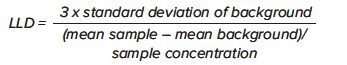As the variation in background increases, the limit of detection also increases. Also like signal-to-noise ratio, it does not take into account signal variation.

### Z’-Factor

The Z’-factor is the standard statistical parameter in the high-throughput screening community for measuring the quality of a screening assay independent of test compounds. It is used as a measure of the signal separation between the positive controls and the negative controls in an assay, and it contains all four parameters that we identified as being useful for measuring and comparing instrument performance.

The Z’-factor is calculated as follows: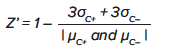where σC+ and σC– are the standard deviations of the positive and negative control groups, respectively, and μC+ and μC– are the means of the positive and negative control groups.

Note that Z’ is not linear and can be made unrealistically small by outliers that skew the standard deviations in either population.

Z’ values are distributed and interpreted as follows:

• \~1 = a perfect assay.
• 0.5 = clear window equal to about half the difference between the positive and the negative controls.
• 0.4 = generally acceptable minimum for assay.
• 0 = overlap between the positive and negative control populations at the 3-sigma level.
• < 0 = substantial overlap.

The significance of Z’ can be understood a little better if we represent it graphically, as in Figure 3.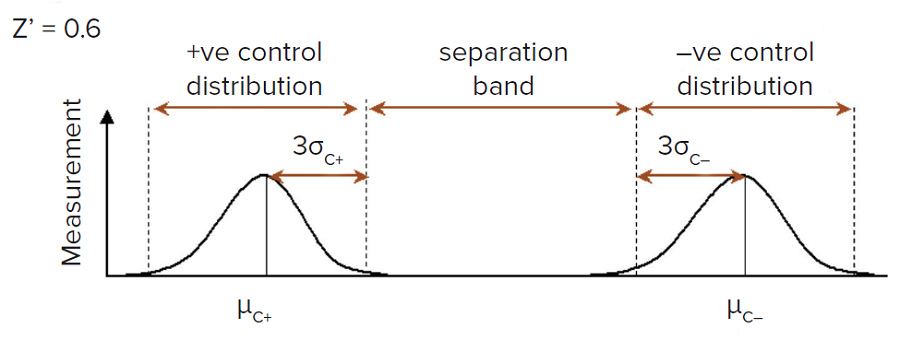Figure 3. Z’-factor as separation between positive and negative controls3

Unlike the signal-to-noise ratio and the limit of detection, the Z’-factor takes into account variation in the signal. Therefore unlike those two metrics, it can distinguish between instruments based on the variation in signal.

Figure 4 shows an example of data from two readers A and B. Intuitively we prefer the data from reader A, because although the variation in the background is higher, there less variation in the signal and thus clear separation between signal and background data. By contrast reader B has smaller variation in background, but much larger variation in signal. This is captured in the values of the various metrics: because Z’ includes signal variation, reader A scores better than reader B on Z’, while reader B scores better than reader A on signal-to-noise and signal-to-background.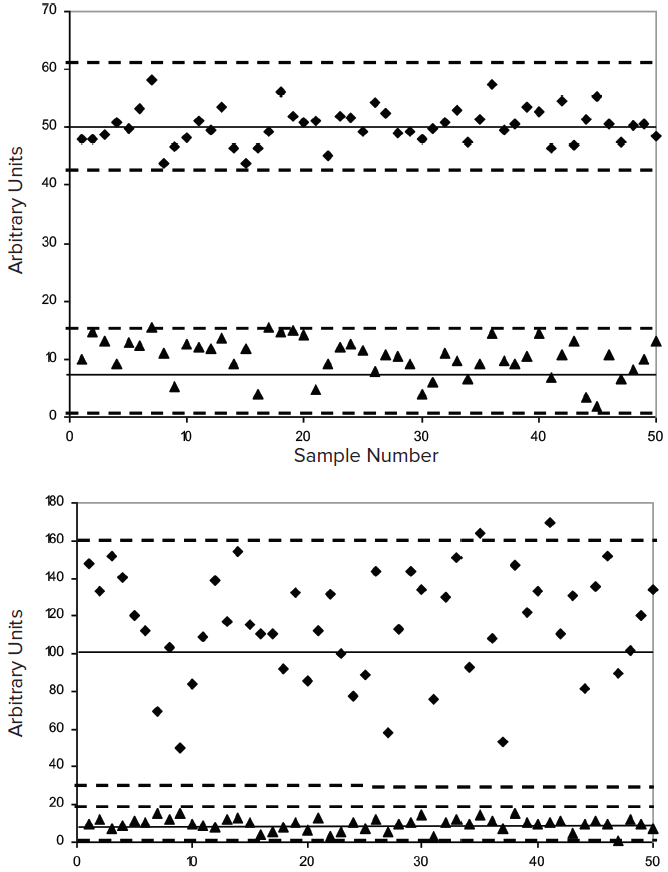Figure 4. Examples of Z’-Factors4. Top: S/B=5; S/N=12; Z’=0.5. Bottom: S/B=12; S/N=32; Z’=0.1.

1. Zhang JH, Chung TD, Oldenburg KR, “A Simple Statistical Parameter for Use in Evaluation and Validation of High Throughput Screening Assays.” J Biomol Screen. 1999;4(2):67–73.
2. Zhang et al., ibid., “Because the S/B ratio does not contain any information regarding data variation, its inappropriateness in evaluation of an assay should be obvious.”
3. Following Figure 4 in Zhang et al., ibid.
4. Following Figure 1 in Zhang et al., ibid.